Search IntMath
Close

450+ Math Lessons written by Math Professors and Teachers

5 Million+ Students Helped Each Year

1200+ Articles Written by Math Educators and Enthusiasts

Simplifying and Teaching Math for Over 23 Years

# The object with finite volume but infinite surface area

By Murray Bourne, 22 Mar 2018

Here's an interesting paradox. I have a hollow object which has infinite height. I can fill the object with paint, but if I try to cover the surface with that paint, there won't be enough.

How can this be?

## Gabriel's horn

According to some, the archangel Gabriel (shared by Judaism, Islam and Christianity) is expected to blow a horn to indicate the last days are upon us.

The mathematical paint paradox involves the volume and surface area of a 3D object resembling Gabriel's horn in this picture.## Generating the object

Consider the hyperbola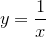: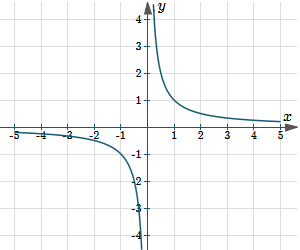We choose the right-hand portion (to avoid the discontinuity at x = 0) and plot the graph starting at x = 1. We consider the (infinite) shaded area to the right of the dashed line, below the curve and above the x-axis.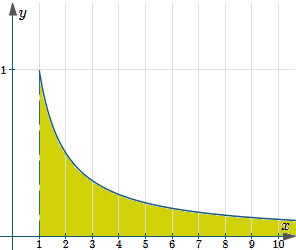We rotate that area in 3 dimensions around the x-axis and end up with an (infinitely long) horn-like object.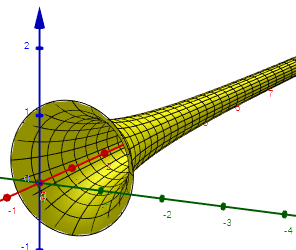### Volume of the horn

Let's now find the volume of the object we've drawn. We use the formula for the volume of a solid of revolution: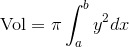where:

a and b are the lower and upper x-limits of the shape

y is the curve we rotate around the axis, in this case 1/x

So in this case, our volume will be as follows (I'm retaining b as the upper limit since we can't substitute - we'll need to consider limits instead):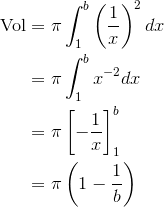As b gets larger and larger, the final fraction in our answer gets smaller and smaller, so the volume is a finite quantity, π.

In mathematical notation, we would write this as: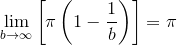### Surface area

Next, let's consider the surface area of the horn.

The formula for the surface area of a solid of revolution is: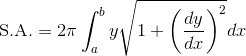where

a and b are the lower and upper x-limits of the shape

y is the curve we rotate around the axis

dy/dx is the derivative of y

In this case, we have: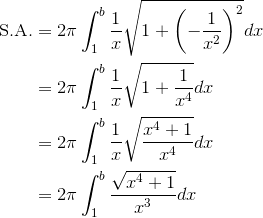If we consider the value of the fraction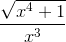as x gets very large, we can see it will get very close to 1/x (but will be just a little bit bigger than it).

So we can conclude that: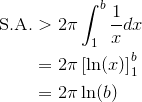Now as b gets larger and larger, this quantity also gets larger. That is, it is an infinitely large quantity.

Here's the graph of our two solutions (in the variable b) - the magenta (pink) one is the volume V = π (1 − 1/b), while the green one is the surface area, S.A. = 2π ln(b). We can see the volume is tending to a limit (π), while the surface area just keeps on getting bigger.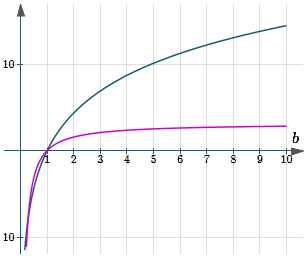So if we try to paint the outer surface with our π liters of paint, it will quickly run out.

## What's going on?

So how can it be that we have a finite volume, but an infinite surface area?

One way of looking at it is to recall how a volume of solid of revolution is actually calculated using integral calculus. We are finding the sum of the volumes of an infinite number of disks, radius y (for different values of x). The disks have area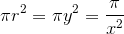and infinitesimal height dx. So each disk has volume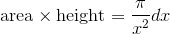The sum of an infinite number of such disks will converge, since this general sum converges: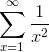### The dispute

The paradox about an object having finite volume but infinite surface area caused a lot of dispute regarding the nature of infinity among mathematicians of the 17th century, including Galileo and Wallis. Such paradoxes are a great way to get us thinking!

### 6 Comments on “The object with finite volume but infinite surface area”

1. Christian Luca says:

A great post, indeed!

I was wondering, though, with regard to the surface area of the solid of revolution, as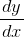foris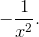When the derivative is squared, it's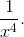The end result would be the same, though, as above as x approaches infinity, with the expression in the integrand being,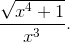2. Vijay singh says:

Good evening
A very nice problem with a great answer.
I would like to add or I must say that I want comments on these lines particularly which I am going to write. If we see this problem practically then
Actually the volume we get after integrating is tending to that volume as length is infinitesimally large. So it will hardly make any difference in volume whereas it's surface area will keep on increasing.

3. Murray says:

@Christian: Thanks for pointing out the error, which I have fixed in the post. I didn't question myself as the end result was the same, as you said!

4. Jan says:

So what would happen if someone were to hold the Horn vertically and fill it up with π litres of paint? Would the inside surface of the Horn get covered in paint entirely?

5. Murray says:

@Jan: Excellent question! Counter-intuitive it may be, but I suspect not. Anyone else like to weigh in?

6. Maritime universities in Chennai says:

Great article about the object with finite volume but infinite surface area...

### Comment Preview

HTML: You can use simple tags like <b>, <a href="...">, etc.

To enter math, you can can either:

1. Use simple calculator-like input in the following format (surround your math in backticks, or qq on tablet or phone):
a^2 = sqrt(b^2 + c^2)
(See more on ASCIIMath syntax); or
2. Use simple LaTeX in the following format. Surround your math with $$ and $$.
$$\int g dx = \sqrt{\frac{a}{b}}$$
(This is standard simple LaTeX.)

NOTE: You can mix both types of math entry in your comment.